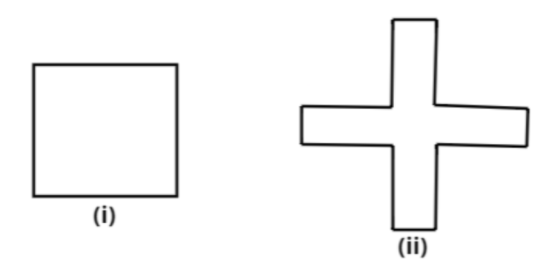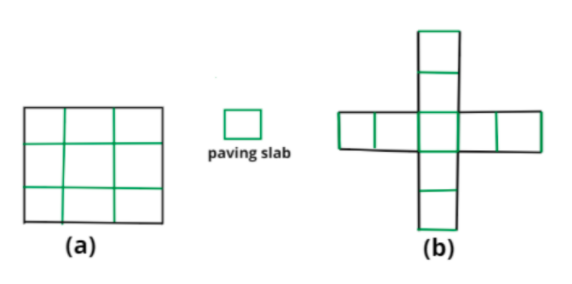Courses
Courses for Kids
Free study material
Free LIVE classes
MoreLIVE
Join Vedantu’s FREE Mastercalss

# Avneet buys 9 square paving slabs, each with a side of $\dfrac{1}{2}$m.He lays them in the form of a square. What is the perimeter of his arrangement? (see figure)Verified
360.9k+ views
Hint: Each side of the figure (i) has three slabs and each strip of figure (ii) has two slabs.Now, solving both parts of the question.
$i)$ As we can clearly see from the figure (a),
There are three slabs on each side of the square.
And we know that side length of the slab is,
$\Rightarrow$Side length of slab $= \;\dfrac{1}{2}$m
$\Rightarrow$So, side length of the square will be $= \;3*\dfrac{1}{2}{\text{m}} = \dfrac{3}{2}{\text{m}}$
Now, we know that the perimeter of all squares is 4$n$.
where $n$ is the side length of the square.
$\Rightarrow$So, perimeter of the figure (i) will be $4*\dfrac{3}{2}{\text{m}} = 6{\text{m}}$.
Hence, the perimeter of figure (i) is 6m

Now, solving other part,
$ii)$ As we can clearly see from the figure (b) that,
Each strip of the figure has two slabs and there are four strips.
And we know that side length of the slab is,
$\Rightarrow$Side length of slab $= \;\dfrac{1}{2}$m
$\Rightarrow$So, perimeter of one strip will be $\left( {\dfrac{1}{2}*2} \right) + \dfrac{1}{2} + \left( {\dfrac{1}{2}*2} \right) = \dfrac{5}{2}{\text{m}}$
And the given figure (ii) has four strips. So, perimeter of the given figure will be,
$\Rightarrow$Perimeter of the given figure (ii) is $4*\dfrac{5}{2}{\text{m}} = 10{\text{m}}$
Hence, the perimeter of figure (ii) is 10m.

Note: Whenever we are asked to find the perimeter of the given figure then the easiest way to find perimeter is by dividing the sides of the figure into side lengths of small slabs whose side length is given, and then add them up to get the total perimeter of the figure.
Last updated date: 19th Sep 2023
Total views: 360.9k
Views today: 7.60k